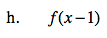### Home > AC > Chapter 12 > Lesson 12.4.2 > Problem12-76

12-76.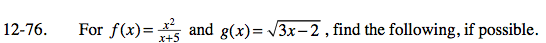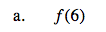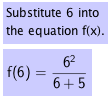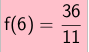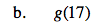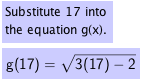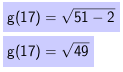g(17) = 7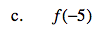f(−5) = undefined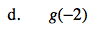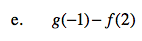Substitute −1 into the equation g(x) and 2 into the equation f(x).

Subtract the second answer from the first.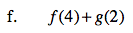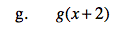$\textit{g}( \textit{x} + 2 ) = \sqrt{3\textit{x} + 4}$